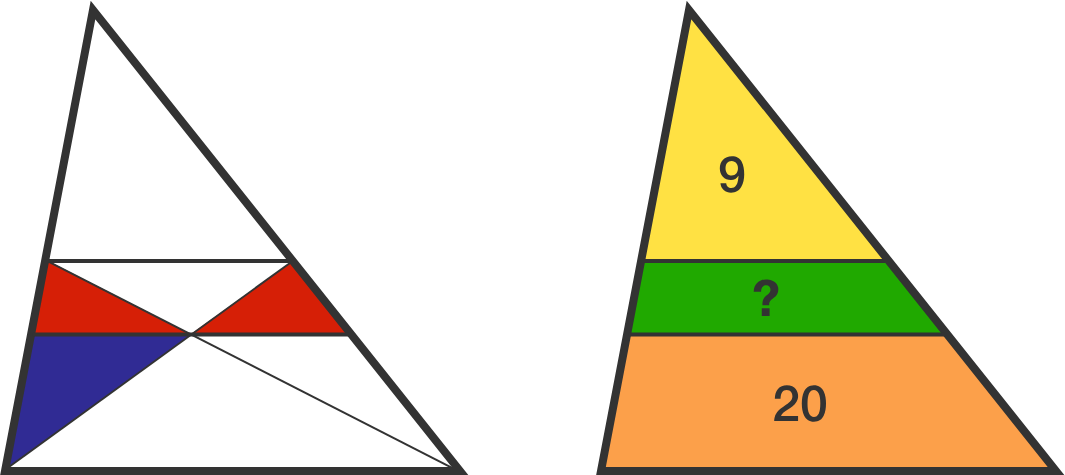# Triangle Riddle

Two lines are drawn within a triangle such that they are both parallel to the triangle's base. The lower of the two lines passes through the intersection point of the diagonals of the trapezoid containing the upper of the two lines and the base of the triangle. The resulting blue triangle, in the diagram below left, has an area equal to the combined area of the $2$ red triangles.If, in the image above right, the number 9 indicates the area of the yellow triangle and the number 20 indicates the area of the orange trapezoid, what is the area of the green trapezoid?

×